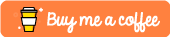Bit Manipulation For Beginners

In this tutorial we will be taking a look at bit manipulation and how you can use it to optimize some of the different parts of your systems when you are programming.

In this tutorial we will be using Python 3.6 in order to demonstrate some of the concepts covered.

Shift Left

By shifting left we are essentially multiplying our original number by 2 * the number of times we shift left.

>>> 16 << 1 # 16 shifted left once = 16 * 2
32
>>> 16 << 2 # 16 shifted left twice = 16 * (2 * 2)
64

Let’s now look at the binary representation for these numbers and how shifting left affects them.

10000 # 16 in binary
10000 << 1 # shift left once
100000 # Returns 32 in binary, we have shifted one bit to the left

10000 # 16 in binary
10000 << 2 # shift left twice
1000000 # returns 64 in binary, we have shifted 2 bits to the left

Shift Right

It should be noted that there are two distinct types of shift right. These are arithmetic shift rights and logical shift rights.

Arithmetic Shift Right

Arithmetic shift rights essentially perform a division on whatever number was put into it. If we performed an arithmetic shift right on the value 16 in Python and shifted it right 1 then our output would be 8. If we shifted right twice our output would be 4 as we are essentially dividing by 4.

>>> 16 >> 1
8
>>> 16 >> 2
4

Let’s take a look at the binary representation of these numbers:

10000 # 16 in binary
10000 >> 1 # 16 / 2
01000 # 01000 = 8 in binary

If we were to shift right twice on an odd number we would see the following:

1001 # 9 in binary
1001 >> 1 # 9 / 2
0100 # Output is 4 in binary. It has rounded down

Bit Logical Operators

In this section of the tutorial we are going to take a look at the logical operators that can be used in conjunction with your bits.

Bitwise And

Bitwise and will return a 1 if both values to the left and right of our & operator are 1. This results in the following output when we try it across various different inputs.

>>> 1 & 1
1
>>> 1 & 0
0
>>> 0 & 1
0
>>> 0 & 0
0

Bitwise Or

Bitwise Or can be done using the | operator in Python and will return a 1 if either or both of our values are 1.

>>> 0 | 1
1
>>> 1 | 0
1
>>> 0 | 0
0
>>> 1 | 1
1

Bitwise XOR

A Bitwise exclusive or (XOR) can be achieved using the ^ operator. This will return the following results:

>>> 1 ^ 1
0
>>> 1 ^ 0
1
>>> 0 ^ 1
1
>>> 0 ^ 0
0

Complex Bit Manipulation

Now that we have covered the basics in Bit manipulation we can start to look at the more complex tasks such as setting a bit, clearing a bit or getting a bit. The vast majority of these tasks can be performed by first creating what we would call a bit mask and then using this with one of the logical operators that we have previously covered.

A bit mask typically looks something like 0010000 and we can use this for doing things like setting, getting or clearing a bit in the 5th bit position. If this doesn’t make sense right now hopefully after the next few examples it will start to become clearer.

Setting a Bit

def set_bit(position, binary):
# Create a bit mask based on the
# position passed in
# produces '10000' if we pass in position=4
# our bit in the '4th' position is set to 1
bit_mask = 1 << position
# return our binary string or-ed with our mask
return bit_mask | binary

# This should return 16
print(set_bit(4, 00000000))